You can Download Samacheer Kalvi 12th Maths Book Solutions Guide Pdf, Tamilnadu State Board help you to revise the complete Syllabus and score more marks in your examinations.

## Tamilnadu Samacheer Kalvi 12th Maths Solutions Chapter 12 Discrete Mathematics Ex 12.3

Choose the correct or the most suitable answer from the given four alternatives.

Question 1.
A binary operation on a set S is a function from …….
(a) S ➝ S
(b) (S × S) ➝ S
(c)S ➝ (S × S)
(d) (S × S) ➝ (S × S)
Solution:
(b) (S × S) ➝ S

Question 2.
Subtraction is not a binary operation in
(a) R
(b) Z
(c) N
(d) Q
Solution:
(c) N
Hint:
For example 2, 5 ∈ N but 2 – 5 = 3 ∉ NQuestion 3.
Which one of the following is a binary operation on N ?
(a) Subtraction
(b) Multiplication
(c) Division
(c) All the above
Solution:
(b) Multiplication

Question 4.
In the set R of real numbers ‘*’ is defined as follows. Which one of the following is not a binary operation on R ?
(a) a * b = min (a.b)
(b) a * b = max (a, b)
(c) a * b = a
(d) a * b = ab
Solution:
(d) a * b = ab
Hint:
Since -2, 1/2 ∈ R , but (-2)1/2 ∉ R.

Question 5.
The operation * defined by a * b = $$\frac{a b}{7}$$ is not a binary operation on ……….
(a) Q+
(b) Z
(c) R
(c) C
Solution:
(b) Z
Hint:
Since 3, 5 ∈ Z, but $$\frac{3 \times 5}{7} \notin$$ Z.Question 6.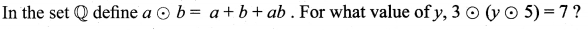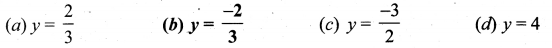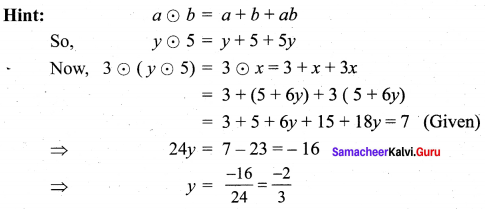Solution:
(b) y = $$\frac{-2}{3}$$

Question 7.
If a * b = $$\sqrt{a^{2}+b^{2}}$$ on the real numbers then * is ……..
(a) commutative but not associative
(b) associative but not commutative
(c) both commutative and associative
(d) neither commutative nor associative
Let, a, b ∈ R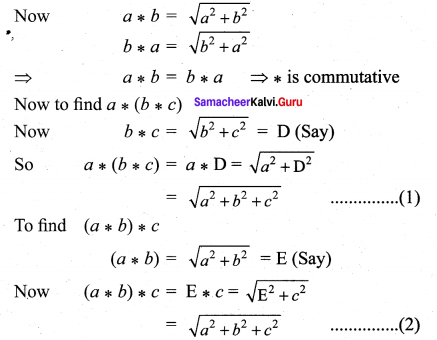(1) = (2) = * is associative
So * is both commutative and associative
Solution:
(c) both commutative and associative

Question 8.
Which one of the following statements has the truth value T ?
(a) sin x is an even function.
(b) Every square matrix is non-singular
(c) The product of complex number and its conjugate is purely imaginary
(d) $$\sqrt{5}$$ is an irrational number
Solution:
(d) $$\sqrt{5}$$ is an irrational number

Question 9.
Which one of the following statements has truth value F ?
(a) Chennai is in India or $$\sqrt{2}$$ is an integer
(b) Chennai is in India or $$\sqrt{2}$$ is an irrational number
(c) Chennai is in China or $$\sqrt{2}$$ is an integer
(d) Chennai is in China or $$\sqrt{2}$$ is an irrational number
Solution:
(c) Chennai is in China or $$\sqrt{2}$$ is an integer

Question 10.
If a compound statement involves 3 simple statements, then the number of rows in the truth table is ……….
(a) 9
(b) 8
(c) 6
(d) 3
Solution:
(b) 8
Hint:
(i.e.) 23 = 8

Question 11.
Which one is the inverse of the statement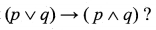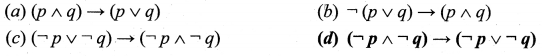Solution:
(a) $$(\neg p \wedge \neg q) \rightarrow(\neg p \vee \neg q)$$

Question 12.
Which one is the contrapositive of the statement $$(p \vee q) \rightarrow r$$?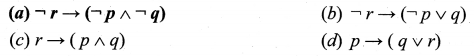Solution:
(a) $$\neg r \rightarrow(\neg p \wedge \neg q)$$Question 13.
The truth table for $$(p \wedge q) \vee \neg q$$ is given below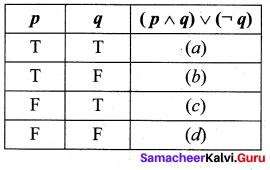Which one of the following is true?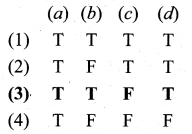Hint: The truth table for $$(p \wedge q) \vee \neg q$$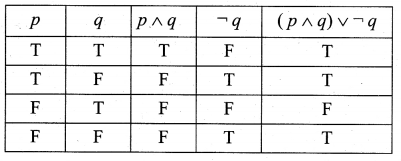Solution:
(3) T T F T

Question 14.
In the last column of the truth table for $$\neg(p \vee \neg q)$$ the number of final outcomes of the truth value ‘F’ are
(a) 1
(b) 2
(c) 3
(d) 4
Hint:
The truth table for $$\neg(p \vee \neg q)$$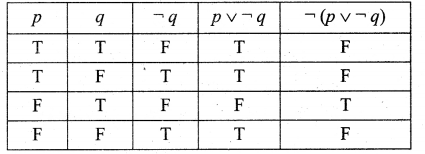Solution:
(c) 3

Question 15.
Which one of the following is incorrect? For any two propositions p and q, we have …….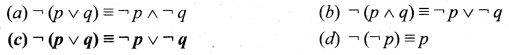Solution:
(c) $$\neg(p \vee q) \equiv \neg p \vee \neg q$$

Question 16.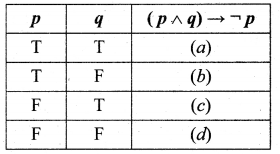Which of the following is correct for the truth $$(p \wedge q) \rightarrow \neg p$$ ?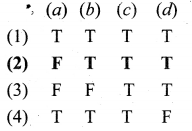Hint: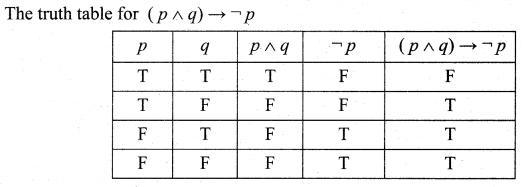Solution:
(2) F T T TQuestion 17.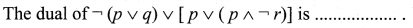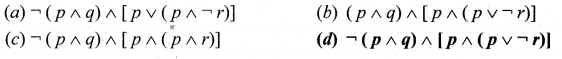Solution:
(d) $$\neg(p \wedge q) \wedge | p \wedge(p \vee \neg r)]$$

Question 18.
The proposition $$p \wedge(\neg p \vee q)]$$ is ……..
(a) a tautology
(b) a contradiction
(c) logically equivalent to $$p \wedge q$$
(d) logically equivalent to $$p \vee q$$
Solution:
(c) logically equivalent to $$p \wedge q$$

Question 19.
Determine the truth value of each of the following statements:
(a) 4 + 2 = 5 and 6+ 3 = 9
(b) 3 + 2 = 5 and 6 + 1 = 7
(c) 4 + 5 = 9 and 1 + 2 = 4
(d) 3 + 2 = 5 and 4 + 7 = 11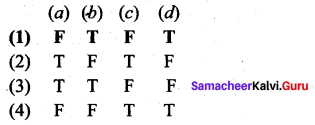Solution:
(1) F T F TQuestion 20.
Which one of the following is not true?
(a) Negation of a negation of a statement is the statement itself.
(b) If the last column of the truth table contains only T then it is a tautology.
(c) If the last column of its truth table contains only F then it is a contradiction
(d) If p and q are any two statements then p ⟷ q is a tautology.
Solution:
(d) If p and q are any two statements then p ⟷ q is a tautology.

### Samacheer Kalvi 12th Maths Solutions Chapter 12 Discrete Mathematics Ex 12.3 Additional Problems

Choose the correct or the most suitable answer from the given four alternatives.

Question 1.
Which of the following are statements?
(i) May God bless you
(ii) Rose is a flower
(iii) milk is white
(iv) 1 is a prime number
(a) (i), (ii), (iii)
(b) (i), (ii), (iv)
(c) (i), (iii), (iv)
(d) (ii), (iii), (iv)
Hint:
Sentence (ii), (iii) and (iv) are statements
(ii) Rose is a flower – True
(iii) Milk is white – True
(iv) 1 is a prime number
∴ (ii), (iii), (iv) are statements
(i) May god bless you. This statement can not be assigned True or False.
∴ (i) is not a statements
Solution:
(d) (ii), (iii), (iv)

Question 2.
If a compound statement is made up of the three simple statements, then the number of rows in the truth table is …….
(a) 8
(b) 6
(c) 4
(d) 2
Hint:
The number of rows in truth table = 2n = 23 = 8
Solution:
(a) 8Question 3.
If p is T and q is F, then which of the following have the truth value T? ………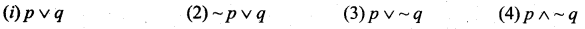(a) (i), (ii), (iii)
(b) (i), (ii), (iv)
(c) (i), (iii), (iv)
(d) (ii), (iii), (iv)
Hint:
p is T then ~p is F
q is F then ~ q is T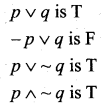Solution:
(c) (i), (iii), (iv)

Question 4.
The number of rows in the truth offimg6 is ……..
(a) 2
(b) 4
(c) 6
(d) 8
Hint:
Number of simple statements given is 2. i.e., p and q.
Number of rows in the truth table of $$\sim[p \wedge(\sim q)]$$ = 22 = 4
Solution:
(b) 4

Question 5.
The conditional statement p ➝ q is equivalent to ……..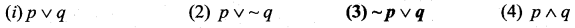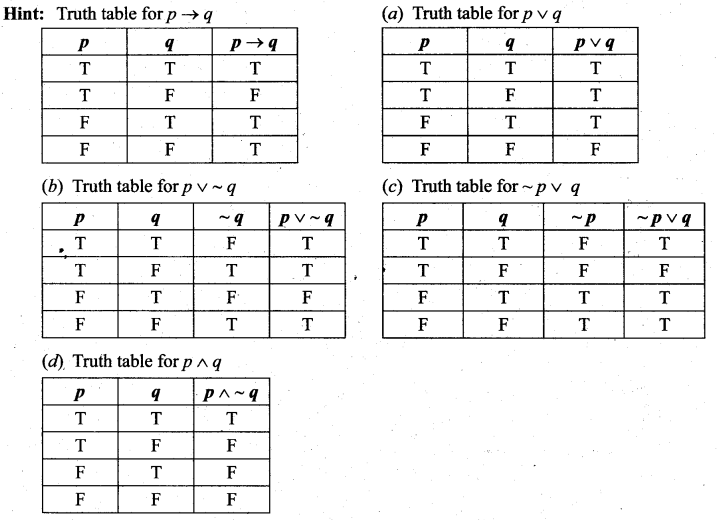The truth table for p ➝ q and $$(\sim p \vee q)$$ having the last column identical.
∴ p ➝ q is equivalent to $$(\sim p \vee q)$$
Solution:
(3) $$\sim p \vee q$$

Question 6.
Which of the following is a tautology?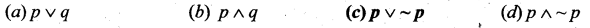Solution:
(c) $$\boldsymbol{p} \vee \sim \boldsymbol{p}$$
Hint:
A statement is said to be a tautology if the last column of its truth table contains only T.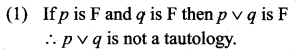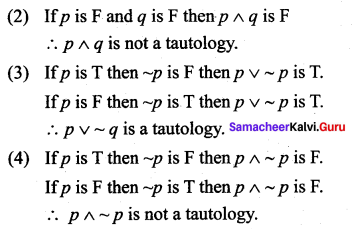Question 7.
In the set of integers with operation * defined by a * b = a + b – ab, the value of 3 * (4 * 5) is ……..
(a) 25
(b) 15
(c) 10
(d) 5
Hint:
a * b = a + b – ab
3 * (4 * 5) = 3 * (4 + 5 – 4(5))
= 3 * (9 – 20)
= 3 * (-11)
= 3 + (-11) – 3(-11)
= 3 – 11 + 33
= -8 + 33 = 25
Solution:
(a) 25

Question 8.
In the multiplicative group of cube root of unity, the order of $$\omega^{2}$$ is ……
(a) 4
(b) 3
(c) 2
(d) 1
Solution:
(b) 3
Hint: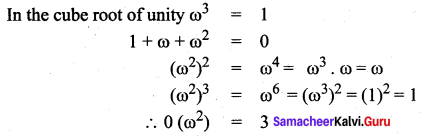Question 10.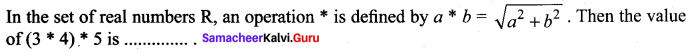(a) 5
(b) 5$$\sqrt{2}$$
(c) 25
(d) 50
Hint: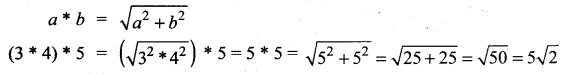Solution:
(b) 5$$\sqrt{2}$$Question 11.
The order of -i in the multiplicative group of 4th roots of unity is ……..
(a) 4
(b) 3
(c) 2
(d) 1
Hint:
The roots of fourth roots of unity are 1, -1, i, -i
The identity element is 1
(-i)4 = i4 = 1
Order of (-i) = 4.
Solution:
(a) 4